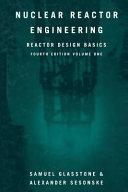### Computational Methods in Modern Physics

Data is displayed for academic year: 2023./2024.

#### Course Description

Different computing methods used for simulations in contemporary physics problems are presented through four topics. Ray tracing in curved spacetime: Spacetime. Curved spacetime. Metric. Path length. Riemannov tensor. Einstein s equation and spherically symmetric solutions. Light propagation in curved spacetime. Numerical solutions of differential equation. Ray-tracing. Gravitational lens. Application of machine learning to event classification in high energy physics: Introduction to ground-based gamma-astronomy: observed objects and instruments. Data acquisition and analysis chain. Event reconstruction. Problem of signal separation in the presence of high levels of noise. Application of random forest algorithm to the gamma-hadron separation problem in high energy physics. Atomic bonds in molecules and solids: quantum-mechanical model and classical approximation. Crystal lattice. Material surface adsorption: chemisorption and physisorption. Van der Waals material layers (graphen as an example). Finding optimal structure by quantum-mechanical simulation and/or using classical approximation. Calculation of ionizing radiation shields by fast engineering methods. Green's function method for point and distributed radiation sources. Build-up factor for gamma radiation inside shields. Green's function for removing fast neutrons in water. Diffusion approximation of the neutron transport equation and some of its applications.

#### Study Programmes

[FER3-EN] Computing - study
Elective Courses (6. semester)
[FER3-EN] Electrical Engineering and Information Technology - study
Elective Courses (6. semester)

#### Learning Outcomes

1. Describe the curved spacetime and light trajectories in the curved spacetime.
2. Apply ray tracing technique to optics problems.
3. Describe the crystal lattice and interatomic forces.
4. Apply the concept of force and energy to finding the optimum configuration of a system.
5. Explain the concept of short and long range interactions.
6. Identify quantitatively abrupt structural change in a system.
7. Apply a machine learning algorithm to a classification problem.

#### Forms of Teaching

Lectures

Introduction to physics of the topic

Independent assignments

Work on projects

Laboratory

Help for work on projects

Continuous Assessment Exam
Homeworks 0 % 100 % 0 % 0 %
Class participation 75 % 0 % 75 % 0 %
Exam: Oral 100 %

#### Week by Week Schedule

1. High-energy physics events
2. High-energy physics events, Application of machine learning to event classification
3. Application of machine learning to event classification
4. Metric in curved spacetime
5. Geodesic equation, Metric in curved spacetime
6. Geodesic equation, Tracing photon trajectories
7. Tracing photon trajectories
8. Midterm exam
9. Van der Waals force interactions
10. Van der Waals force interactions, Graphene layers
11. Graphene layers
13. Neutron transport equations and applications.
14. Neutron transport equations and applications.
15. Final exam

#### Literature

(.), V. Šips, I. Rendulić: Uvod u fiziku čvrstog stanja,
(.), General Relativity, MIT OpenCourseWare https://ocw.mit.edu/ans15436/ZipForEndUsers/8/8-962-spring-2006/8-962-spring-2006.zip,Samuel Glasstone, Alexander Sesonske (1994.), Nuclear Reactor Engineering: Reactor Design Basics, Springer
(.), Albert, J., et al. (2008). Implementation of the random forest method for the imaging atmospheric Cherenkov telescope MAGIC. Nuclear Instruments and Methods in Physics Research A, 588, 424,

#### General

ID 223383
Summer semester
5 ECTS
L1 English Level
L1 e-Learning
45 Lectures
0 Seminar
0 Exercises
13 Laboratory exercises
0 Project laboratory